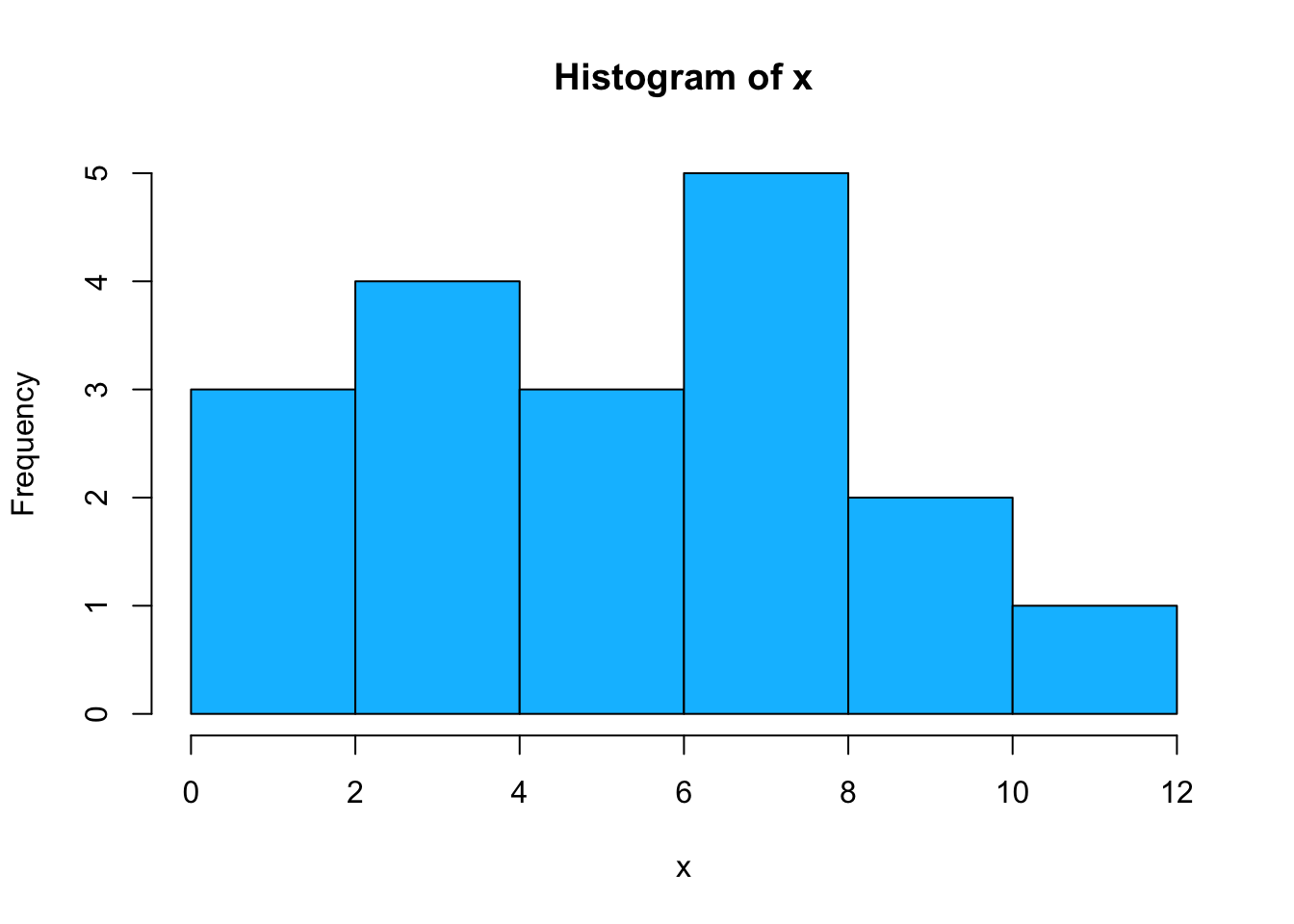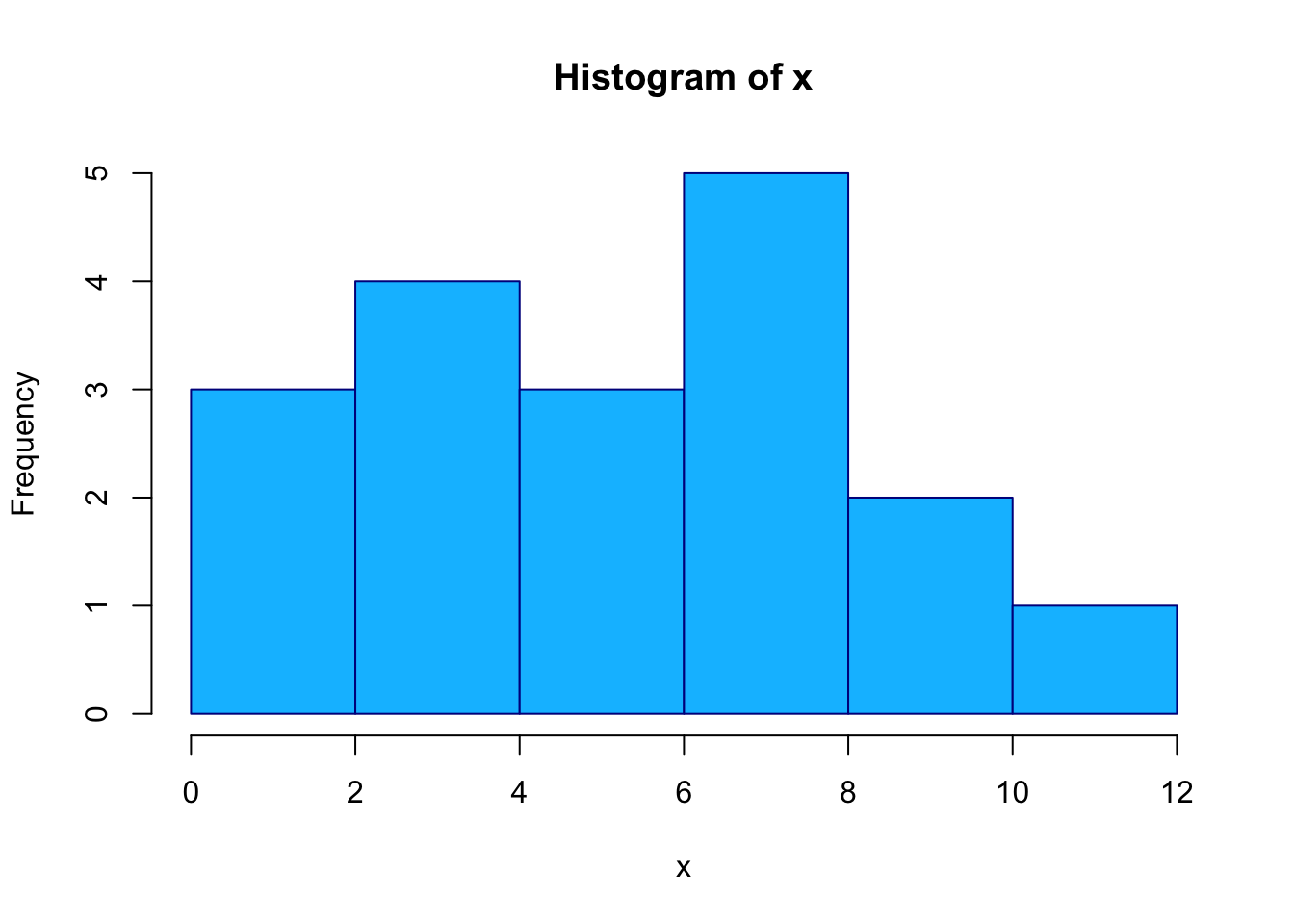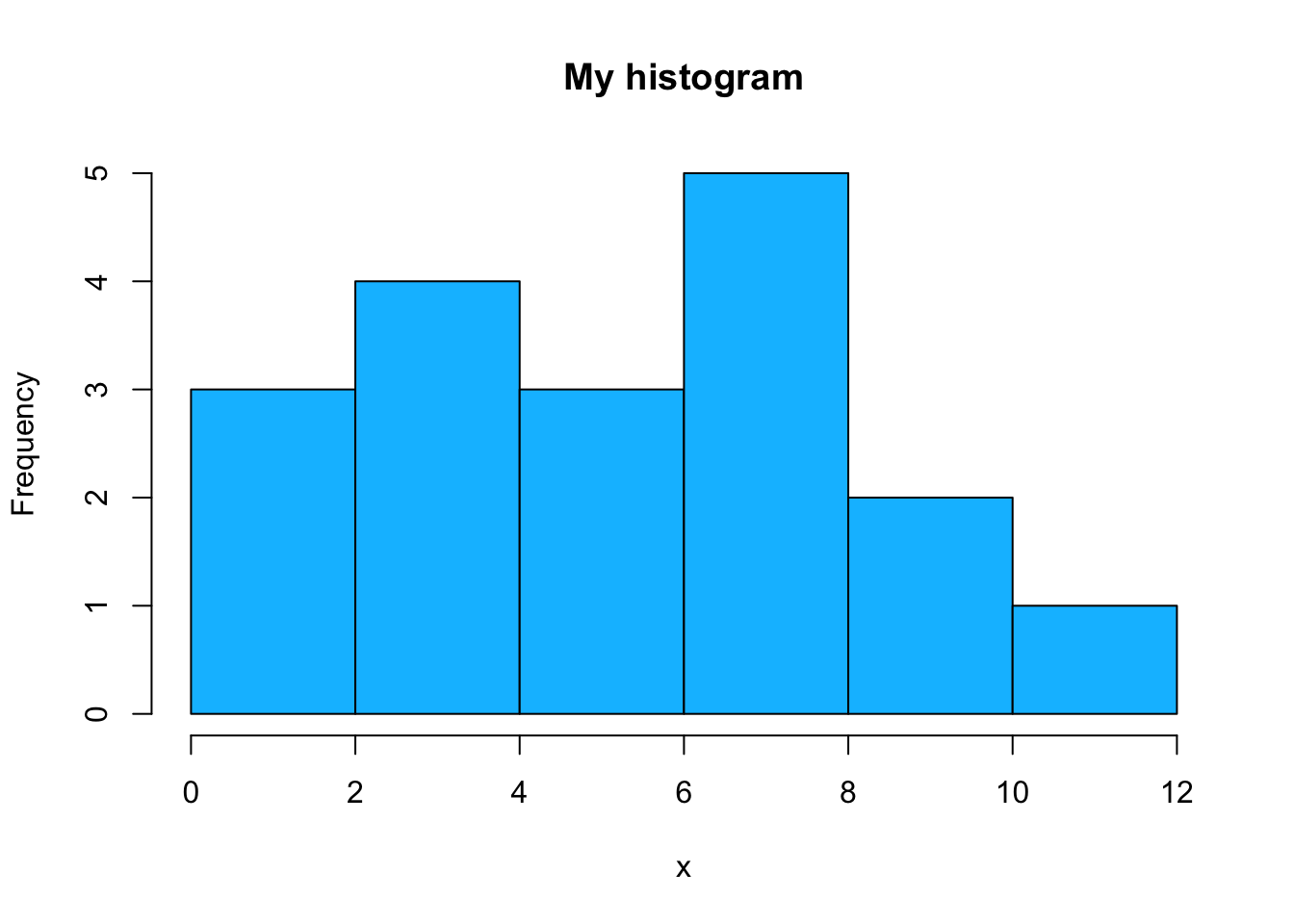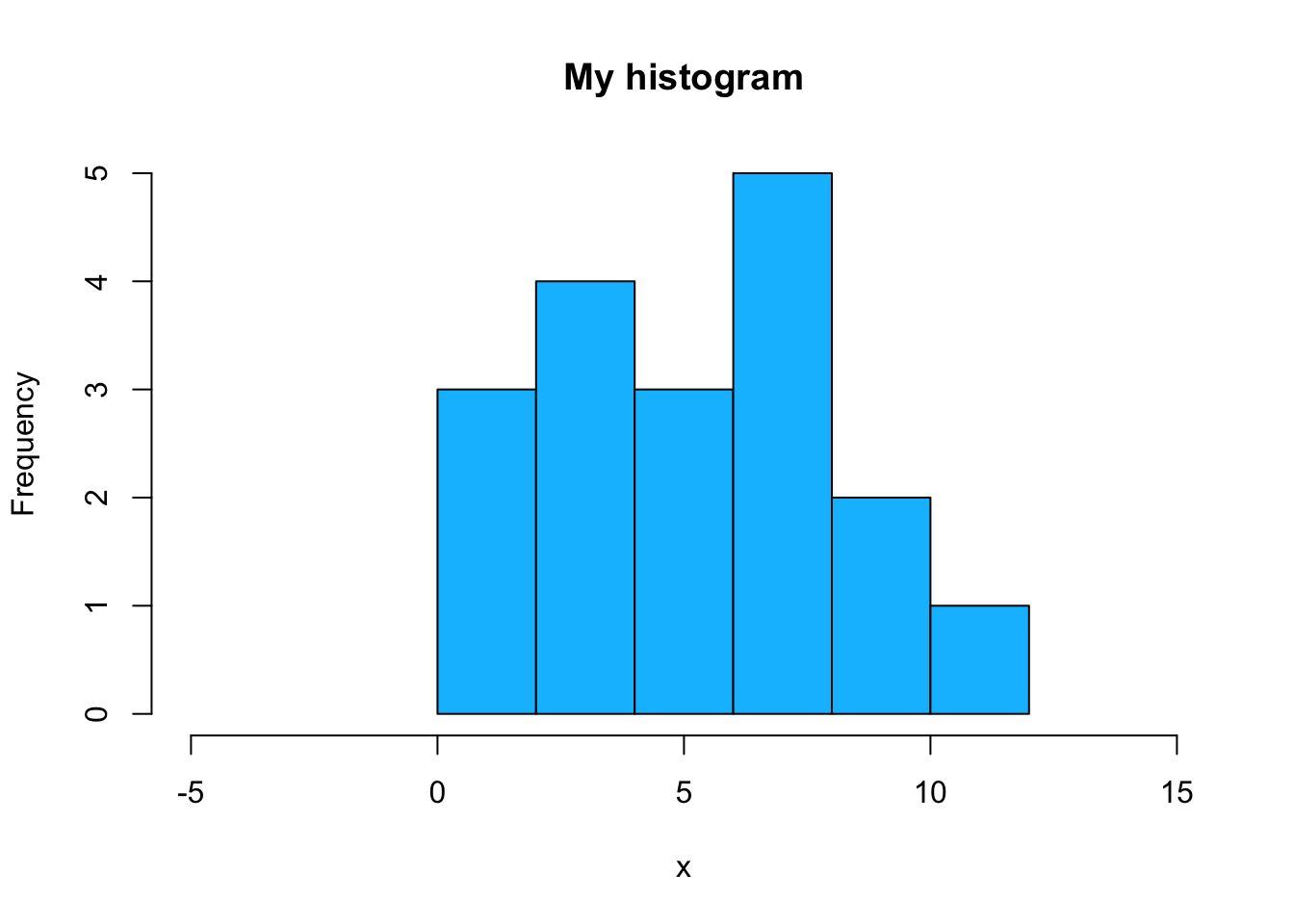## More on basic graphs in R

### Experimenting with histograms

Let’s create a vector that we will use for experimenting with graphics:

``````x <- c(2, 8, 10, 1, 2, 12, 10, 7, 7, 7, 8,
3, 3, 3, 3, 5, 6, 5)``````

First, let’s plot a basic histogram and change its color:

``hist(x, col = "deepskyblue")``Multiple colors are usually used for bar charts, for plots of categorical variables instead of quantitative ones. One color corresponds to one category, so different categories are represented by bars in different colors. In histograms all bins stand for the same, for absolute frequencies. That’s why, histograms are never multicolored.

We can change a border color as well:

``````# make a border darkblue
hist(x, col = "deepskyblue", border = "darkblue")````````hist(x, col = "deepskyblue", main = "My histogram")``We can specify the limits of a horizontal or of a vertical axis in graphs. For example, we can restrict x-axis to include values from 5 to 15:

``````# xlim - x-axis
hist(x, col = "deepskyblue",
main = "My histogram",
xlim = c(-5, 15))``````The same can be done for y-axis, let’s set it from 0 to 8:

``````# y-lim - y-axis
hist(x, col = "deepskyblue",
main = "My histogram",
xlim = c(-5, 15),
ylim = c(0, 8))``````